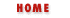home | O'Reilly's CD bookshelfs | FreeBSD | Linux | Cisco | Cisco Exam

# 4. Expressions and Operators

Expressions and operators are fundamental to most programming languages. This chapter explains how they work in JavaScript. If you are familiar with C, C++, or Java, you'll notice that expressions and operators in JavaScript are very similar, and you'll be able to skim this chapter quickly. If you are not a C, C++, or Java programmer, this chapter will teach you what you need to know about expressions and operators in JavaScript.

## 4.1 Expressions

An expression is a "phrase" of JavaScript that a JavaScript interpreter can evaluate to produce a value. Simple expressions are constants (e.g., string or numeric literals) or variable names, like these:

```1.7                               // a numeric literal
"Oh no!  We're out of coffee!"    // a string literal
true                              // a Boolean literal
null                              // the literal null value
i                                 // the variable i
sum                               // the variable sum
```

The value of a constant expression is simply the constant itself. The value of a variable expression is the value that the variable refers to.

These expressions are not particularly interesting. More complex (and interesting) expressions can be created by combining simple expressions. For example, we saw that 1.7 is an expression and i is an expression, so the following is also an expression:

```i + 1.7
```
The value of this expression is determined by adding the values of the two simpler expressions. The plus sign in this example is an operator that is used to combine two expressions into a more complex expression. Another operator is - which is used to combine expressions by subtraction. For example:

```(i + 1.7) - sum
```
This expression uses the - operator to subtract the value of the sum variable from the value of our previous expression i + 1.7. JavaScript supports a number of other operators, besides + and -, which we'll learn about in the next section.Data Type Wrapper ObjectsOperator Overview